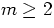Next: Sky Images Up: Proposed Conventions Previous: Proposed Conventions

## 4.2.1 Improved Axis Descriptions

The original FITS Paper (Wells, Greisen and Harten 1981), assumed that the units of the axes would be the basic SI units appropriate to the CTYPEn axes. But the choice of units may not be obvious. GC propose that a new keyword be reserved for such cases:

CUNITn (floating) is the units in which both CRVALn and CDELTn for axis n are expressed.

Units identified using this keyword should be chosen using the principles defined in section 3.1.1.4 and must conform to all the requirements in that section.

An axis may be described by more than one label. For example, a spectrum may be described by frequency, wavelength, and velocity, only one of which can be linear. GC propose reserving the following additional keywords:

• CmYPEn (floating) is label m for axis n.
• CmNITn (floating) gives the units for axis n when label m is used.
• CmPIXn (floating) is the reference point of axis n when label m is used.
• CmVALn (floating) is the coordinate value of the reference point on axis n when label m is used.

The values of m may run from 2-9; the original CTYPEn, CRPIXn, CRVALn, and CDELTn keywords are used for m=1. Note that the reference pixel need not be the same for all descriptions of an axis. The values of a given pixel in the different units must be consistent, e.g., 1420.4 MHz and 21.1 cm. There are no defaults assumed for; all of these keywords should be specified.Next: Sky Images Up: Proposed Conventions Previous: Proposed Conventions